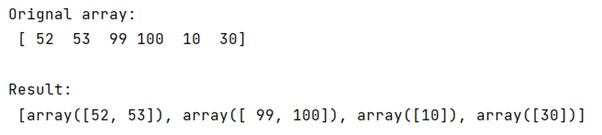# How to find the groups of consecutive elements in a NumPy array?

Learn, how to find the groups of consecutive elements in a NumPy array in Python?
Submitted by Pranit Sharma, on January 12, 2023

NumPy is an abbreviated form of Numerical Python. It is used for different types of scientific operations in python. Numpy is a vast library in python which is used for almost every kind of scientific or mathematical operation. It is itself an array which is a collection of various methods and functions for processing the arrays.

## Finding the groups of consecutive elements in a NumPy array

Suppose we are given a NumPy array containing some randomly ordered integer values but also some values are consecutive to each other. We need to find the groups of consecutive elements in this array.

For this purpose, it would be better to define a function so that we can perform the check on each element of the array.

We will use numpy.where() and return the group of those elements which are consecutive.

Let us understand with the help of an example,

## Python code to find the groups of consecutive elements in a NumPy array

```# Import numpy
import numpy as np

# Creating numpy array
arr = np.array([52,53,99,100,10,30])

# Display original array
print("Orignal array:\n",arr,"\n")

# defining a function to check values
def fun(data):
return np.split(data, np.where(np.diff(data) != 1)+1)

# Calling function
res = fun(arr)

# Display result
print("Result:\n",res)
```

Output:What's New (MCQs)

Top Interview Coding Problems/Challenges!

IncludeHelp's Blogs

Languages: » C » C++ » C++ STL » Java » Data Structure » C#.Net » Android » Kotlin » SQL
Web Technologies: » PHP » Python » JavaScript » CSS » Ajax » Node.js » Web programming/HTML
Solved programs: » C » C++ » DS » Java » C#
Aptitude que. & ans.: » C » C++ » Java » DBMS
Interview que. & ans.: » C » Embedded C » Java » SEO » HR
CS Subjects: » CS Basics » O.S. » Networks » DBMS » Embedded Systems » Cloud Computing
» Machine learning » CS Organizations » Linux » DOS
More: » Articles » Puzzles » News/Updates